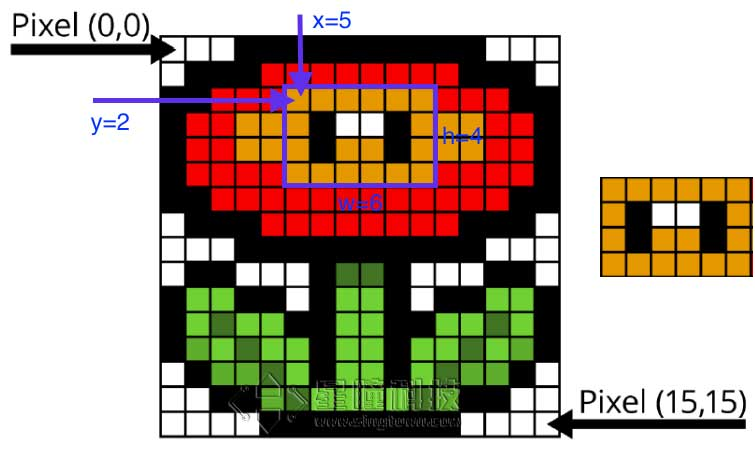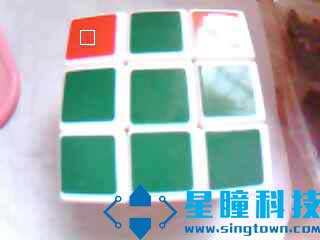# 使用图像的统计信息

## ROI感兴趣的区域roi的格式是(x, y, w, h)的tupple.

• x:ROI区域中左上角的x坐标
• y:ROI区域中左上角的y坐标
• w:ROI的宽度
• h:ROI的高度

## Statistics

``````image.get_statistics(roi=Auto)
``````

``````img.get_statistics(roi=(0,0,10,20))
``````

• statistics.mean() 返回灰度的平均数(0-255) (int)。你也可以通过statistics获得。

• statistics.median() 返回灰度的中位数(0-255) (int)。你也可以通过statistics获得。

• statistics.mode() 返回灰度的众数(0-255) (int)。你也可以通过statistics获得。

• statistics.stdev() 返回灰度的标准差(0-255) (int)。你也可以通过statistics获得。

• statistics.min() 返回灰度的最小值(0-255) (int)。你也可以通过statistics获得。

• statistics.max() 返回灰度的最大值(0-255) (int)。你也可以通过statistics获得。

• statistics.lq() 返回灰度的第一四分数(0-255) (int)。你也可以通过statistics获得。

• statistics.uq() 返回灰度的第三四分数(0-255) (int)。你也可以通过statistics获得。

• l_mean，l_median，l_mode，l_stdev，l_min，l_max，l_lq，l_uq，
• a_mean，a_median，a_mode，a_stdev，a_min，a_max，a_lq，a_uq，
• b_mean，b_median，b_mode，b_stdev，b_min，b_max，b_lq，b_uq，

## 举例

``````import sensor, image, time

sensor.reset() # 初始化摄像头
sensor.set_pixformat(sensor.RGB565) # 格式为 RGB565.
sensor.set_framesize(sensor.QVGA)
sensor.skip_frames(10) # 跳过10帧，使新设置生效
sensor.set_auto_whitebal(False)               # Create a clock object to track the FPS.

ROI=(80,30,15,15)

while(True):
img = sensor.snapshot()         # Take a picture and return the image.
statistics=img.get_statistics(roi=ROI)
color_l=statistics.l_mode()
color_a=statistics.a_mode()
color_b=statistics.b_mode()
print(color_l,color_a,color_b)
img.draw_rectangle(ROI)
````````````56 66 51
56 66 55
56 66 51
56 66 51
56 66 51
56 66 51
56 66 51
56 66 51
56 66 51
56 66 51
56 66 51
56 66 51
56 66 51
56 66 51
56 66 51
56 66 51
56 66 55
56 66 51
56 66 51
56 66 51
56 66 51
56 66 51
``````